Duane Nykamp

•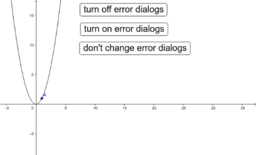test error

Activity

Duane Nykamp

•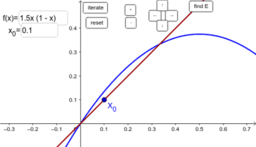Cobweb linear approximations around equilibria

Activity

Duane Nykamp

•The chain rule as multiplying slopes

Activity

Duane Nykamp

•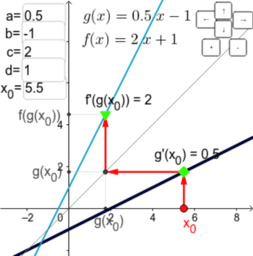The chain rule for linear functions

Activity

Duane Nykamp

•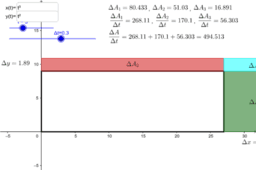Product rule change in area

Activity

Duane Nykamp

•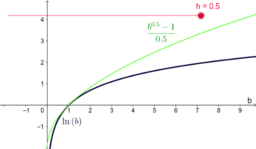Limit of b to the h minus one over h 2

Activity

Duane Nykamp

•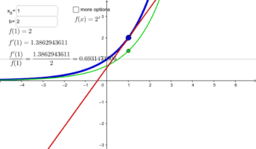The derivative of the exponential function

Activity

Duane Nykamp

•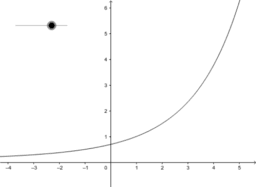Limit of b to the h minus one over h

Activity

Duane Nykamp

•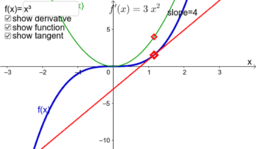The derivative of a function

Activity

Duane Nykamp

•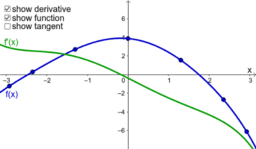Derivative of interpolating polynomial

Activity

Duane Nykamp

•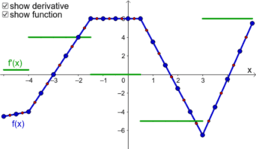The derivative of a piecewise linear function

Activity

Duane Nykamp

•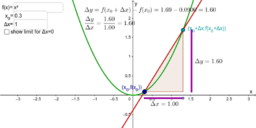The slope of a secant line

Activity

Duane Nykamp

•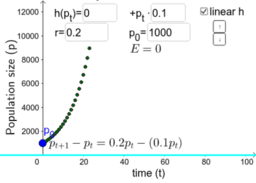The dynamics of a population with variable harvesting

Activity

Duane Nykamp

•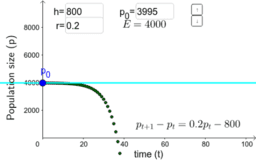The dynamics of a population with harvesting fixed number

Activity

Duane Nykamp

•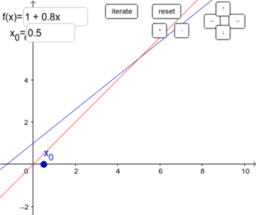Visualizing function iteration via cobwebbing and plot

Activity

Duane Nykamp

•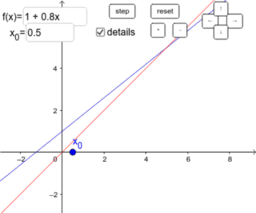Visualizing function iteration via cobwebbing

Activity

Duane Nykamp

•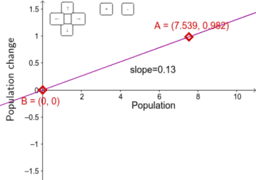Fitting a linear model to population change

Activity

Duane Nykamp

•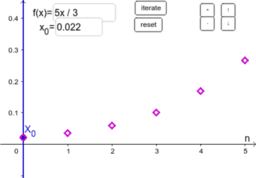Function iteration using initial bacteria growth model

Activity

Duane Nykamp

•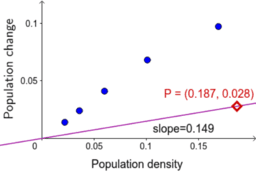Fitting a linear model to bacteria population change

Activity

Duane Nykamp

•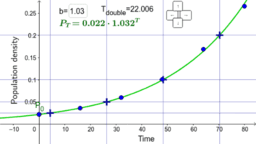Doubling time and half life with bacteria data

Activity

Duane Nykamp

•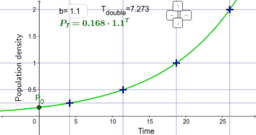Doubling time and half life

Activity

Duane Nykamp

•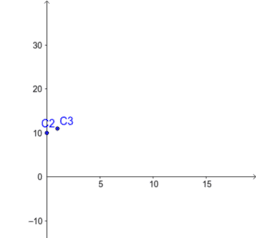Activity

Duane Nykamp

•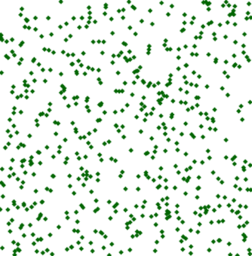Evolution of a bistable population

Activity

Duane Nykamp

•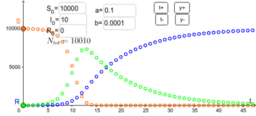Activity

Duane Nykamp